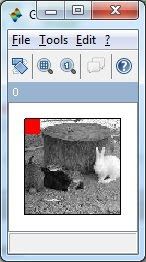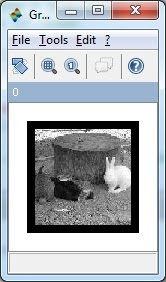### Drawing Shapes by Overwriting Pixel Value

1. How to highlight certain portion on an image?

The basic concept of highlighting part of an image is “Overwriting Pixel Value” on the image. We start from the basic idea on how to mask portion of image with blank sub-image (black color sub-image). Figure below shows the image with the size of 128x128x3 in which 3 represents RGB. (In grayscale image 3 layers are the same). The second image at the right hand site shows a black sub-image is placed at the upper left of the original image. The Scilab code to perform the operation are as follow:S2 = S;
S2(:,:,2) = S;
S2(:,:,3) = S; // Create a gray-scale RGB Image
S = S2; // Save a copy of image
ShowColorImage(S2,'0');Sblack = uint8(zeros(20,20,3));
S2 = S;
S2(1:20,1:20,:) = Sblack;
ShowColorImage(S2,'0');2. How to create a color mask rather than black color mask?

Since the 3 layers of image matrix represent RGB layers of the image, we can create the red color mask using following command, and the results are shown as shown.

Sred = Sblack;
Sred(:,:,1)=255;
S2 = S;
S2(1:20,1:20,:) = Sred;
ShowColorImage(S2,'0');3. How to create a transparent mask?

Simple enough, just play with the values R value, and perform the image addition rather than overwriting the value as follow:

Sred = Sblack;
S2 = S;
S2(1:20,1:20,1) = 255;
ShowColorImage(S2,'0');

4. Finally, how to create an outline for the image?

linelength = 10;
Sblack = uint8(zeros(128+linelength*2,128+linelength*2,3));
S2 = Sblack;
S2((1+linelength):(\$-linelength),(1+linelength):(\$-linelength),:)=S;
ShowColorImage(S2,'0');